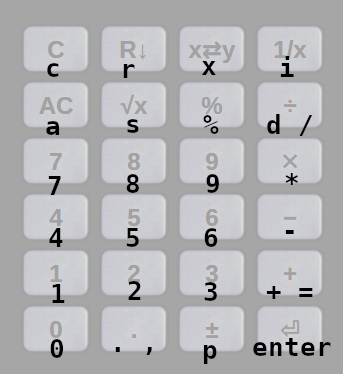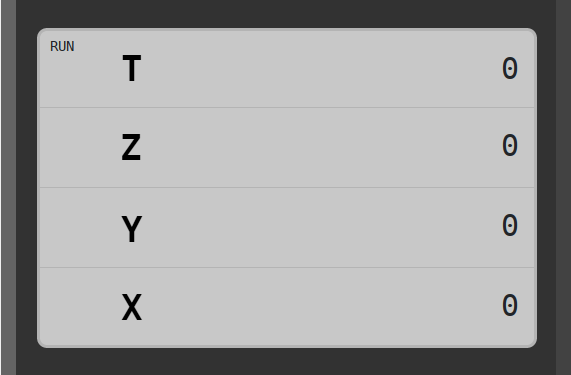# Artinu - Reverse Polish Notation Calculator

## 1. Introduction

Reverse Polish Notation is a method of notation of mathematical expressions that allows simple calculations to be performed without the need of using brackets - thanks to the use of a stack. This method has been popularized by Hewlett Packard, which has been successfully using it in its calculators for many years.
The Artinu calculator is a simple application, written in JavaScript, available online. It was developed for educational purposes. RPN calculators available on the market, may overwhelm the novice user with an excess of buttons and available functions. Our calculator allows only basic actions to be performed, so that the user can easily grasp the idea of operations on the stack without having to worry about other issues.
The use of RPN comes with great educational value - learning operations at the stake is a very good introduction to computer science and programming. An additional motivation for creating this application is the fact that currently in Poland, during the secondary school-leaving exam, young people can only use the simplest calculators. It seems that producing on its basis an inexpensive, physical device with similar properties for school purposes, should not pose any major difficulties.

## 2. Performing basic calculations

Unlike a classical device, the RPN calculator requires that you first enter the numbers on which you perform the action and then enter the action itself.
Example 1 - adding two numbers:
In order to make an addition: 5+9, enter in sequence: 5 9 +. The result will appear at the bottom of the display.
Example 2 - square root:
To calculate the square root of 25, use the keys: 2 5 . The result will appear at the bottom of the display.

## 3. Description of the keys

• Number keys: 0 ... 9.
• Decimal separator: ..
• Change the sign of the number to the opposite one: ±.
• "Enter" approves the entered number without performing an arithmetic operation:.
• Keys for two-argument operations: +, -, ×, ÷.
• Keys for unary operations: , %, 1/x.
• Stack operation keys:
• R↓ (roll) - All values on the stack except for the X register (at the very bottom), are moved to the cell below. The register X replaces the contents of the T register (at the very top).
• x⇄y (swap) - swaps the values in the X and Y registers with places.
• Delete keys:
• C (clean) - deletes the entire X register, or the last digit entered, depending on whether the entered number has already been confirmed by "enter" or not.
• AC (all clean) - will reset all register values and restore the calculator to its initial state.

## 4. Shortcuts for PC

The following keyboard shortcuts are available for PCs and other devices equipped with a physical keyboard:Additionally, the "h" and "F1" keys display a help file.

## 5. Stack operations

If the description contained in this part seems too tedious to the readers, after learning the names of registers - one can go straight to the examples. You can also learn it by experimenting with the calculator itself.

### 5.1. Registers

The calculator contains four memory cells called registers. They are usually called: X, Y, Z, T. In most of the available non-graphic RPN calculators, only two registers are displayed at once: X and Y (one register in the older machines). Our calculator displays all 4 of them, which makes it easier to capture the principle of its operation. The picture below shows the positions of the individual registers:Initially, the values of all registers are initialized with zeros. After entering a number, we can choose one of the following:
1. Confirming it with the "enter" key: .
2. Performing one of the arithmetic actions: +, -, ×, ÷, , 1/x, %.
3. Performing one of the stack operations: R↓, x⇄y.
If we confirm a number with the "enter" key, it will appear simultaneously in two registers: X and Y. If, on the other hand, we finish entering a number with one of the arithmetic operations, the calculator will behave as follows:
1. If the operation is binary (using two arguments), it will be executed on X and Y registers, and the result will appear in the X register.
2. If the operation is unary (using one argument), it will be executed on the entered number and its result will appear in the X register.

### 5.2. Shifting registers

#### 5.2.1. Binary arithmetic operation

The execution of a two-argument operation, changes the content of registers according to the following rules:
• X - contains the result of the operation,
• Y - contains the previous contents of the Z register,
• Z - contains the previous contents of the T register,
• T - is zeroed.

#### 5.2.2. Unary arithmetic operation

The execution of a single-argument operation, causes the following change in the content of registers:
• X - contains the result of operation,
• the other registers remain unchanged.

#### 5.2.3. R↓ Operation

The execution of the R↓ operation (roll), causes the following change in the content of registers:
• X - contains the previous contents of the Y register,
• Y - contains the previous contents of the Z register,
• Z - contains the previous contents of the T register,
• T - contains the previous contents of the X register,

## 6. Example - commission on the sale of an apartment

The problem:
We buy an apartment worth 380000 EUR. The agent demands a commission of 2.5%. VAT of 23% is added to the commission. We want to know the total value of the commission plus VAT.
Solution:
• We enter the price of the apartment: 3 8 0 0 0 0 (x = 380000, y = 380000)
• We calculate the commission percentage: 2 . 5 % (x = 9500, y = 380000)
• We calculate the VAT on the commission: 2 3 % (x = 2185, y = 9500, z = 380000)
• We add VAT to the commission: + (x = 11685, y = 380000)
After the calculation is completed, the X register contains the commission together with VAT (11685 EUR), and the Y register - the original price of the apartment.

## 7. Example - total resistance

The problem:
We want to calculate the total resistance for two resistors connected in parallel:using a formula: $$\frac{1}{R} = \frac{1}{R_1} + \frac{1}{R_2}$$
The input data:
$$R_1 = 1.5\ \mathrm{k}Ω$$ $$R_2 = 2.3\ \mathrm{k}Ω$$
Solution:
• 1 . 5 1/x - the inverse of the first resistance value is stored in the X register ($$x \approx 0.67$$),
• 2 . 3 1/x - the inverse of the secondary resistance value is stored in the X register, and the Y register now contains the value calculated in the previous step ($$x \approx 0.43, y \approx 0.67$$),
• + 1/x - we add both stored values and count the inverse, resulting in: $$R \approx\ 0.91\ \mathrm{k}Ω$$

## 8. Example - Pythagorean theorem

The problem:
We want to find the length of the hypotenuse of right-angled triangle, having the length of its legs equal: $$a = 5.5,$$ $$b = 6.7,$$ using Pythagorean theorem: $$c^2 = a^2 + b^2$$.
Solution:
Because our simple calculator has no $$x^2$$ operator, the following property of RPN calculators is used here: after approving a number with the "enter" key, it is placed in both X and Y registers together.
• 5 . 5 × - after this operation, the X register contains $$a^2$$
• 6 . 7 × - after this operation, the X register contains $$b^2$$ and the Y register contains $$a^2$$.
• + - finally we get the result $$c \approx 8.67$$